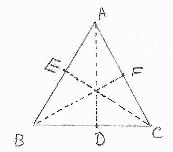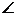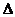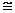# If the altitudes of a triangle ABC are equal,then prove that triangle is equilateral.Given, AD, BE and CF are the attitudes drawn on sides BC, CA and AB of Δ ABC such that AD = BE = CF

Area of Δ ABC =× BC × AD =× AB × CF =× CA × BE (Area of Δ =× Base × Correspondence attitude)

∴ BC × AD = AB × CF = CA × BE

⇒ BC = AB = CA (AD = BE = CF)

Hence, ΔABC is an equilateral triangle.

• 61

Prove tht the bisectors of the angles of a linear pair are right angles

• -1

prove tht the measure of each angle of an equalateral triangle is 60

solve this one nt the above one plzzz i got  tht

• -3
 Steps Statement Reason1 Consider triangles BEC and BFC 2 EC=BF Equal altitudes(given) 3BEC=BFC = 900 BE and BF are Altitudes 4 BC is common 5BECBFC RHS postulate 6ABC =BCA Corresponding angles 7 Consider theADB andADC 8ADB=ADC = 900 AD is Altitude 9 AD is common 10ABC =BCA Step 6 11ADBADC ASA postulate 12 AB =AC Corresponding sides are equal 13 BC= AC Similarly we can proveBFCBFA 14 AB=AC=BC Step 12,13

• 11
What are you looking for?